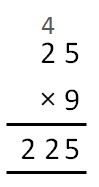Fractions to decimals

Chapter 8 Class 6 Decimals
Concept wise

#### Write 3/5 as decimal

For decimals,

we need 10 in the denominator

So,

3/5 = 3/5 × 2/2

= 6/10

= 0.6

We  can do the same for  other numbers as well

#### Write 1/2 as decimal

1/2 = 1/2 × 5/5

= 5/10

= 0.5

∴ 1/2 = 0.5

#### Write 9/4 as decimal

9/4

We know that

4 × 25 = 100

So,

9/4 = 9/4 × 25/25

= 225/100

= 2.25#### Write 81/1000 as decimal

81/1000

= 0081/1000

= 0.081

Get live Maths 1-on-1 Classs - Class 6 to 12

### Transcript

Write 𝟑/𝟓 as decimal For decimals, we need 10 in the denominator So, 3/5 = 3/5 × 2/2 = 6/10 = 0.6 ∴ 3/5 = 0.6 We can do the same for other numbers as well Write 𝟏/𝟐 as decimal 1/2 = 1/2 × 5/5 = 5/10 = 0.5 ∴ 1/2 = 0.5 Write 𝟗/𝟒 as decimal 9/4 We know that 4 × 25 = 100 So, 9/4 = 9/4 × 25/25 = 225/100 = 2.25 Write 𝟖𝟏/𝟏𝟎𝟎𝟎 as decimal 81/1000 = 0081/1000 = 0.081